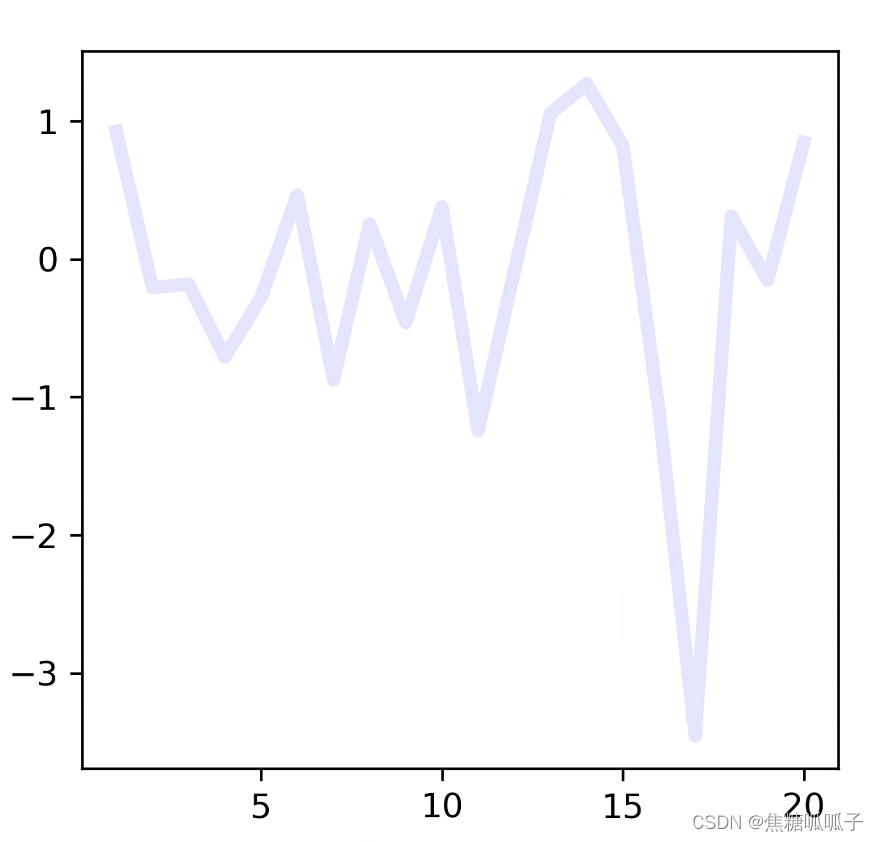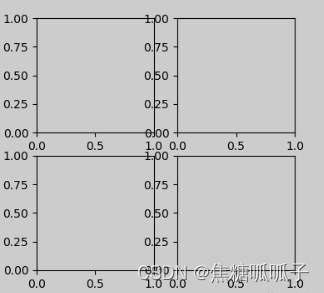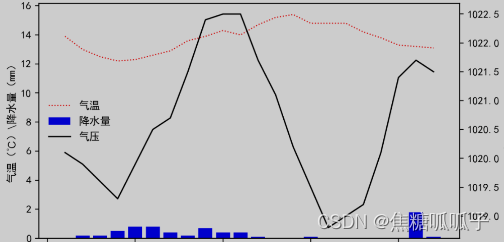# 前言

## 一、基础命令

``plt.plot(x,y,lw=,ls=，c=, alpha=, label=)``

x：x坐标的数据

y：y坐标的数据

lw：指定线条宽度

ls：指定线条样式，ls='-'为实线，ls='–'为虚断线，ls='-.'为点虚线，ls=':'为虚线

c：指定线条颜色，c='r'为红色，c='k'为黑色，c='y'为黄色

alpha：指定线条透明度，值越小越透明

label：指定线条的含义

``````#导入库
import matplotlib.pyplot as plt
import numpy as np
#设定画布。dpi越大图越清晰，绘图时间越久
fig=plt.figure(figsize=(4, 4), dpi=300)
#导入数据
x=list(np.arange(1, 21))
y=np.random.randn(20)
#绘图命令
plt.plot(x, y, lw=4, ls='-', c='b', alpha=0.1)
plt.plot()
#show出图形
plt.show()
#保存图片
fig.savefig("画布")``````## 二、根据Excel数据绘图

``````# 导包
import pandas as pd
# 读取excel文件

``````...
# 第一步绘制画布
fig=plt.figure(figsize=(7, 4), dpi=200)
# 第二步添加绘图区.
# subplot命令是在画布上添加一个绘图区，括号里的内容转述为汉字为：“创建一个一行一列的绘图区（一行一列就只有一个绘图区），ax1是第一个绘图区，facecolor用来设置画布背景颜色，默认为白色
ax1 = fig.add_subplot(111, facecolor='green')
``````

``````ax1=fig.add_subplot(221)## 三、合并一幅图的x（或y）坐标轴以及添加图例legend()``````
fig=plt.figure(figsize=(7,4),dpi=200)        # 新建画布
line1,=ax1.plot(times,temps,'r:',lw=1,label='气温')    # 创建折线
bar1 =ax1.bar(times,rains,color='b',label='降水量')    # 创建条状
ax2=ax1.twinx()                            # 设置共用x轴
line2,=ax2.plot(times,pressures,'k-',lw=1.2,label='气压')

# legend用来设置图例，还可以添加参数ncol=''，该参数用来设置图例的列数，用于对齐
plt.legend((line1,bar1,line2),('气温','降水量','气压'),loc='center left',frameon=False,framealpha=0.5)
ax1.set_xlabel('时间 \ h')                # 设置x轴
ax1.set_ylabel('气温（℃）\降水量（mm）')  # 设置左侧y轴
ax2.set_ylabel('气压（hPa）')             # 设置右侧y轴
plt.title("----")                         # 设置图的名称
plt.show()``````

## 四、调节字体样式

``````font={'size':30,'color':'red'}
ax.set_xlabel('--',fontdict=font)
ax.set_ylabel('--',fontdict=font)``````

## 五、绘制网格线

``````ax.grid()  # 开启x和y轴的网格
ax.grid(ls='--')   # 开启x和y轴的虚线网格
ax.grid(True,axis='x')   # 开启x轴的网格
ax.grid(True,axis='y')   # 开启y轴的网格``````

## 六、合并两幅图的坐标轴

``````fig,((ax1),(ax2))=plt.subplots(2,1,figsize=(5,5),dpi=200,sharex='all')

## 七、不常用的函数

### 2.set_minor_locator()、set_major_locator()

set_minor_locator用来在主刻度的基础上设置或修改副刻度的大小，set_major_locator用来修改主刻度的单位显示。使用前，必须引入库包：

``import matplotlib.ticker as ticker``

（1）此处设定副刻度为0.1个单位。

``ax1.yaxis.set_minor_locator(ticker.MultipleLocator(0.1))``

（2） 将右侧主刻度设置为每10个单位显示。

``ax2.yaxis.set_major_locator(ticker.MultipleLocator(10))``